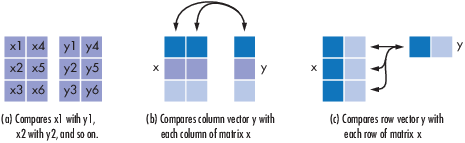Documentation

### This is machine translation

Mouseover text to see original. Click the button below to return to the English version of the page.

# symerr

Compute number of symbol errors and symbol error rate

## Syntax

```[number,ratio] = symerr(x,y) [number,ratio] = symerr(x,y,flg) [number,ratio,loc] = symerr(...) ```

## Description

### For All Syntaxes

The `symerr` function compares binary representations of elements in `x` with those in `y`. The schematics below illustrate how the shapes of `x` and `y` determine which elements `symerr` compares.The output `number` is a scalar or vector that indicates the number of elements that differ. The size of `number` is determined by the optional input `flg` and by the dimensions of `x` and `y`. The output `ratio` equals `number` divided by the total number of elements in the smaller input.

### For Specific Syntaxes

`[number,ratio] = symerr(x,y) ` compares the elements in `x` and `y`. The sizes of `x` and `y` determine which elements are compared:

• If `x` and `y` are matrices of the same dimensions, then `symerr` compares `x` and `y` element by element. `number` is a scalar. See schematic (a) in the figure.

• If one is a row (respectively, column) vector and the other is a two-dimensional matrix, then `symerr` compares the vector element by element with each row (resp., column) of the matrix. The length of the vector must equal the number of columns (resp., rows) in the matrix. `number` is a column (resp., row) vector whose mth entry indicates the number of elements that differ when comparing the vector with the mth row (resp., column) of the matrix. See schematics (b) and (c) in the figure.

`[number,ratio] = symerr(x,y,flg) ` is similar to the previous syntax, except that `flg` can override the defaults that govern which elements `symerr` compares and how `symerr` computes the outputs. The values of `flg` are `'overall'`, `'column-wise'`, and `'row-wise'`. The table below describes the differences that result from various combinations of inputs. In all cases, `ratio` is `number` divided by the total number of elements in `y`.

Comparing a Two-Dimensional Matrix x with Another Input y

Shape of yflgType of Comparisonnumber
Two-dim. matrix `'overall'` (default) Element by element Total number of symbol errors
`'column-wise'`mth column of `x` vs. mth column of `y` Row vector whose entries count symbol errors in each column
`'row-wise'`mth row of `x` vs. mth row of `y` Column vector whose entries count symbol errors in each row
Column vector `'overall'` `y` vs. each column of `x` Total number of symbol errors
`'column-wise'` (default) `y` vs. each column of `x` Row vector whose entries count symbol errors in each column of `x`

Row vector

`'overall'``y` vs. each row of `x` Total number of symbol errors
`'row-wise'` (default) `y` vs. each row of `x` Column vector whose entries count symbol errors in each row of `x`

`[number,ratio,loc] = symerr(...)` returns a binary matrix `loc` that indicates which elements of `x` and `y` differ. An element of `loc` is zero if the corresponding comparison yields no discrepancy, and one otherwise.

## Examples

On the reference page for `biterr`, the last example uses `symerr`.

### Compare Elements of Matrix

Compare Elements of Matrix with Another Matrix

`x = [1,1,3,1;3,2,2,2;3,3,8,3]`
```x = 3×4 1 1 3 1 3 2 2 2 3 3 8 3 ```
`aMatrix = [1,1,1,1;2,2,2,2;3,3,3,3]`
```aMatrix = 3×4 1 1 1 1 2 2 2 2 3 3 3 3 ```
`[number1,ratio1] = symerr(x,aMatrix)`
```number1 = 3 ```
```ratio1 = 0.2500 ```

Compare Elements of Matrix with Row Vector

`x = [1,1,3,1;3,2,2,2;3,3,8,3]`
```x = 3×4 1 1 3 1 3 2 2 2 3 3 8 3 ```
`aRowVector = [1,2,3,1]`
```aRowVector = 1×4 1 2 3 1 ```
`[number2,ratio2] = symerr(x,aRowVector)`
```number2 = 3×1 1 3 4 ```
```ratio2 = 3×1 0.2500 0.7500 1.0000 ```

Compare Elements of Matrix with Column Vector

`x = [1,1,3,1;3,2,2,2;3,3,8,3]`
```x = 3×4 1 1 3 1 3 2 2 2 3 3 8 3 ```
`aColumnVector = [1;2;3]`
```aColumnVector = 3×1 1 2 3 ```
`[number3,ratio3] = symerr(x,aColumnVector)`
```number3 = 1×4 1 0 2 0 ```
```ratio3 = 1×4 0.3333 0 0.6667 0 ```

### Use Alternative Type of Comparison

You can specify alternative comparison methods used by `symerr`. In this example, you use a flag to override the default row-by-row comparison. Notice that number and ratio are scalars.

```format rat; [number,ratio,loc] = symerr([1 2; 3 4],[1 3],'overall')```
```number = 3 ```
```ratio = 3/4 ```
```loc = 2×2 0 1 1 1 ```